# Longitud

La longitud puede medirse en unidades métricas o en unidades del sistema inglés.

La unidad métrica estándar de longitud es el metro , que es un poco mas largo que 3 pies. (La longitud exacta de un metro esta definida por el Buro Internacional de Pesos y Medidas como la longitud de paso viajada por la luz en el vacío durante un período de tiempo corto, llamado 1/299972458 de un segundo. In all likelihood, you will never have to remember this!)

From the meter, we get the rest of the metric units using the standard metric prefixes.

 nanometer(nm)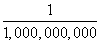of a meter micrometer(um)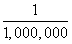of a meter millimeter(mm)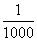of a meter centimeter(cm)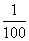of a meter decimeter(dm)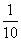of a meter meter(m) dekameter(dam) 10 meters hectometer(hm) 100 meters kilometer(km) 1000 meters Megameter(Mm) 1,000,000 meters Gigameter(Gm) 1,000,000,000 meters Terameter(Tm) 1,000,000,000,000 meters

Metric rulers are usually have centimeters numbered, with smaller marks shown for millimeters.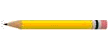The pencil in the figure has a length of 3.4 centimeters, or 34 millimeters.

## U.S. Customary Units

The system for measuring length in the customary system is based on the inch, foot, yard, and mile.Lengths smaller than 1 inch are usually written using fractions of an inch. On a U.S. customary foot ruler, the inch is usually divided into halves, quarters, eigths, and 16ths by progressively smaller markings.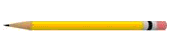In the figure shown, the pencil is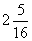inches long.

To convert from one unit to another, we can use unit analysis . For instance, if you want to find the number of inches in 3 miles, you can multiply the quantity 3 miles by the fraction 5280 feet/1 mile and then by the fraction 12 inches/1 foot.

1 mile = 5,280 feet

1 foot = 12 inches

12 inches × 5,280 feet = 63,360 inches in 1 mile

To find the number of inches in 3 miles: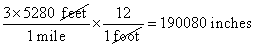(Both these fractions are equal to 1, so you're not changing the original quantity when you multiply.)

Note:

(a) To convert from larger units to smaller units, multiply by the appropriate unit ratio.

(b) To convert from smaller units to larger units, multiply by the reciprocal of the appropriate unit ratio.

(c) Multiplying or dividing by a unit ratio is mathematically equivalent to using a proportion to convert between units of measure .